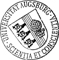## Multidimensional rank-one convexification of incremental damage models at finite strains

• This paper presents computationally feasible rank-one relaxation algorithms for the efficient simulation of a time-incremental damage model with nonconvex incremental stress potentials in multiple spatial dimensions. While the standard model suffers from numerical issues due to the lack of convexity, the relaxation techniques circumvent the problem of non-existence of minimizers and prevent mesh dependency of the solutions of discretized boundary value problems using finite elements. By the combination, modification and parallelization of the underlying convexification algorithms the approach becomes computationally feasible. A descent method and a Newton scheme enhanced by step size control strategies prevents stability issues related to local minima in the energy landscape and the computation of derivatives. Special techniques for the construction of continuous derivatives of the approximated rank-one convex envelope are discussed. A series of numerical experimentsThis paper presents computationally feasible rank-one relaxation algorithms for the efficient simulation of a time-incremental damage model with nonconvex incremental stress potentials in multiple spatial dimensions. While the standard model suffers from numerical issues due to the lack of convexity, the relaxation techniques circumvent the problem of non-existence of minimizers and prevent mesh dependency of the solutions of discretized boundary value problems using finite elements. By the combination, modification and parallelization of the underlying convexification algorithms the approach becomes computationally feasible. A descent method and a Newton scheme enhanced by step size control strategies prevents stability issues related to local minima in the energy landscape and the computation of derivatives. Special techniques for the construction of continuous derivatives of the approximated rank-one convex envelope are discussed. A series of numerical experiments demonstrates the ability of the computationally relaxed model to capture softening effects and the mesh independence of the computed approximations.Author: Daniel Balzani, Maximilian Köhler, Timo NeumeierGND, Malte A. PeterORCiDGND, Daniel PeterseimORCiDGND https://opus.bibliothek.uni-augsburg.de/opus4/99667 Preprint English 2022 Universität Augsburg 2022/11/25 1 28 Mathematisch-Naturwissenschaftlich-Technische Fakultät Mathematisch-Naturwissenschaftlich-Technische Fakultät / Institut für Mathematik Mathematisch-Naturwissenschaftlich-Technische Fakultät / Institut für Mathematik / Lehr- und Forschungseinheit Angewandte Analysis Mathematisch-Naturwissenschaftlich-Technische Fakultät / Institut für Mathematik / Lehrstuhl für Numerische Mathematik 5 Naturwissenschaften und Mathematik / 51 Mathematik / 510 Mathematik Aktuelle Publikationen (noch nicht gedruckt erschienen)Deutsches Urheberrecht